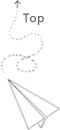# Algorithm(5) hash table and two pointers(2)

### Container With Most Water

Given n non-negative integers a1, a2, …, an, where each represents a point at coordinate (i, ai). n vertical lines are drawn such that the two endpoints of line i is at (i, ai) and (i, 0). Find two lines, which together with x-axis forms a container, such that the container contains the most water.

Note: You may not slant the container.

### Implement strStr()

Returns the index of the first occurrence of needle in haystack, or -1 if needle is not part of haystack.

Given a linked list, determine if it has a cycle in it.

Given a linked list, return the node where the cycle begins. If there is no cycle, return null.

Note: Do not modify the linked list.

Can you solve it without using extra space?

TODO

s1=t=a+nb+c

s2=2t=a+mb+c

### Find the Duplicate Number

Given an array nums containing n + 1 integers where each integer is between 1 and n (inclusive), prove that at least one duplicate number must exist. Assume that there is only one duplicate number, find the duplicate one.

Note:
You must not modify the array (assume the array is read only).【说明不能对数组进行排序】

You must use only constant, O(1) extra space.【说明不能采用时间换空间的方法 建立一个map 或者是新的数组 来记录是否这个元素被访问过】

Your runtime complexity should be less than O(n2).【说明不能采用两层循环的那种遍历方式】

There is only one duplicate number in the array, but it could be repeated more than once.【说明不能采用所有数字再相加 之后再减去 1+2+3+..+n 这种方式 因为可能某个数字重复出现多次】

Credits:
Special thanks to @jianchao.li.fighter for adding this problem and creating all test cases.

### Minimum Size Subarray Sum

Given an array of n positive integers and a positive integer s, find the minimal length of a subarray of which the sum ≥ s. If there isn’t one, return 0 instead.

For example, given the array [2,3,1,2,4,3] and s = 7,
the subarray [4,3] has the minimal length under the problem constraint.

• sum > s 的时候，想要值更小一点，就更新左边的指针
• sum < s 的时候，想让值变得更大一点，就更新右边的指针
• 需要注意的是：边界条件的判定，特别是输入数组为空的时候，否则直接让sum=nums[l]就会可能发生运行时错误，还有再最后的时候，要是没有符合要求的结果，就返回0，这两点自己第一次都没有看出来，事实上这也是判断程序员是否有经验的一个重要方面，面试的时候现场写程序，可能就跪了，边界检查这种东西，真是要时刻牢牢记在心里，要是不提示输入示例的话，还真是不太容易看出来。。。

## 推荐文章